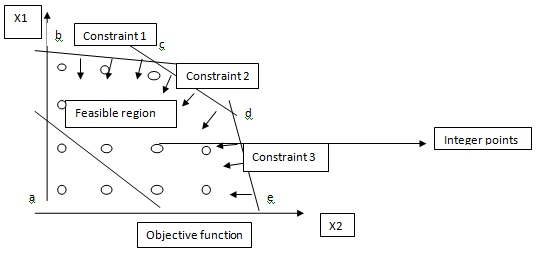# Alternate Optimal Solution

Posted in Operations and Supply Chain Terms, Total Reads: 18505

## Definition: Alternate Optimal Solution

An alternate optimal solution is also called as an alternate optima, which is when a linear / integer programming problem has more than one optimal solution. Typically, an optimal solution is a solution to a problem which satisfies the set of constraints of the problem and the objective function which is to maximize or minimize.

Example:Here, the graphical analysis of a problem is given with set of (< =) constraints and a maximizing objective function. The optimal solution set is a smaller set within the feasible region. Here, the objective function is parallel to cd line segment. Hence, all points (x1, x2) on cd give maximum yield. In such case, there is alternate optimal solution.

Browse the definition and meaning of more terms similar to Alternate Optimal Solution. The Management Dictionary covers over 7000 business concepts from 6 categories.

Search & Explore : Management Dictionary

Similar Definitions from same Category: